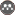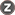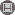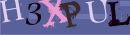Volume 11, Issue 6 (10-2013)                   RSMT 2013, 11(6): 1-14 | Back to browse issues page

BibTeX | RIS | EndNote | Medlars | ProCite | Reference Manager | RefWorks
Send citation to:Rezaei R. Designing a specific upper body anaerobic power test for wrestling. RSMT 2013; 11 (6) :1-14
URL: http://jsmt.khu.ac.ir/article-1-39-en.html
Abstract:   (7230 Views)

Abstract
The aim of this study was designing a specific upper body anaerobic power test for wrestlers and determining validity, reliability and objectivity of the designed test. In order to assess the anaerobic power of wrestlers on the basis of upper body Wingate test, Twenty two wrestlers (age=23/40±3/20 year, height=173/13±6/97 cm, weight=74/55±3/88 kg) of Tehran wrestling team (most of them were Asian champion) were participated in this study. Mean, maximum and minimum of anaerobic power and fatigue indices assessed by both tests. In order to analysis the correlation between power indices and physiological indices (blood lactate and heart rate), Pearson correlation has been used, and the differences between blood lactate after 2 min in both tests assessed. The result indicated that there was significant correlation between maximum (p=0/001 r=0/739), mean (p=0/001 r=0/670) and minimum (p=0/01 r=0/650) of power and fatigue indices (p=0/003 r=0/610) obtained by designed test and Wingate test. The result showed that there was significant correlation between heart rate immediately after doing both tests (p=0/001, r=0/705) and also the result show that there was not significant correlation between blood lactate after 2 min. of doing both tests (p=0/07 r=0/353). Statistical analyses also showed no difference between the blood lactate after 2 min of doing both tests. Analyzing the validity of designed test, revealed that there was significant correlation between max (p=0/000 r=0/840), mean (p=0/000 r=0/861) and minimum (p=0/000 r=0/830) power and fatigue indices (p=0/000 r=772) after performing twice of designed test. In analysis objectively of the designed test, the result revealed that there was significant correlation between max (p=0/000 r=0/832), mean (p=0/000 r=0/858) and minimum (p=0/000 r=0/805) power and fatigue indices (p=0/000 r=0/832) which was done by two different tester. It can be concluded that in respect to Wingate upper body test, the designed test in this study is valid and reliable test to measure the anaerobic power of wrestlers.Rights and permissionsThis work is licensed under a Creative Commons Attribution-NonCommercial 4.0 International License.